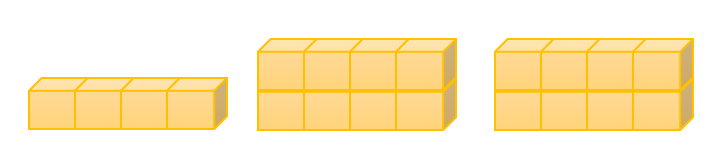Mathematics
Easy

Question

# Lin creates a pattern using the rule “Each layer has 4-blocks”.The number of cubes in the next shape in Lin’s pattern is

## 12    16    20    24Hint:

## The correct answer is: 16

### We are given a pattern with number of cubes. The rule used to make the pattern is “Each layer has four blocks”.The number of cubes in the first shape is 4.The number of cubes in the second shape is (4 + 4) = 8The number of cubes in the third shape is (8 + 4) = 12One layer is added every time. With each layer, 4 blocks are added.So, the number of cubes in the fourth shape will be (12 + 4) = 16.

The main part of the question is the rule used to make the pattern.

### Related Questions to study#### With Turito Foundation.#### Get an Expert Advice From Turito.